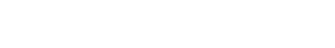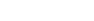# Mix Design of High-Strength Concrete: Methods, Procedure and ExampleReading time: 1 minute

Mix design of high strength concrete is influenced by properties of cement, sand aggregates & water-cement ratio have compressive strength above 40 MPa. To achieve high strength, it is necessary to use lowest possible water-cement ratio, which invariably affects the workability of the mix and necessitates the use of special vibration techniques for proper compaction. In the present state of art, a concrete with a desired 28 day compressive strength of upto 70 MPa can be made with suitably proportioning the ingredients using normal vibration techniques for compacting the concrete mix.Erntroy and Shacklock’s Empirical Graphs: Erntroy and Shacklock have suggested empirical graphs relating the compressive strength to an arbitrary ‘reference number’ for concrete made with crushed granite, coarse aggregates and irregular gravel. These graphs are shown in figure 1 and 2 for mixes with ordinary Portland cement and in figure 3 and 4 for mixes with rapid hardening Portland cement. The relation between water cement ratio and the reference number for 20mm and 10mm maximum size aggregates is shown in figure 5, in which four different degrees of workability are considered. The range of the degrees of workability varying from extremely low to high corresponds to the compacting factor values of 0.65 and 0.95 respectively The relation between the aggregate-cement and water-cement ratios, to achieve the desired degree of workability with a given type and maximum size of aggregate are compiled in table-1 and 2 for two different types of cements. The limitations of these design tables being that they were obtained with aggregates containing 30 percent of the material passing the 4.75 mm IS sieve. Thus, if other ingredients are used suitable adjustments have to be made. Aggregates available at site may be suitably combined by the graphical method to satisfy the above requirement. In view of the considerable variations in the properties of aggregates, it is generally recommended that trial mixes must first be made and suitable adjustments in grading and mix proportions effected to achieve the desired results. Table – 1: Aggregate cement ratio (by weight) required to give four degrees of workability with different water –cement ratios using ordinary Portland cementTable – 2: Aggregate cement ratio (by weight) required to give four degrees of workability with different water –cement ratios using rapid hardening cement## Mix Design of High Strength Concrete -Procedure

1. The mean design strength is obtained by applying suitable control factors to the specified minimum strength.
2. For a given type of cement and aggregates used, the reference number corresponding to the design strength at a particular age is interpolated from figure 1 to 4.
3. The water-cement ratio to achieve the required workability and corresponding to the reference number is obtained from figure 5 for aggregates with maximum sizes of 20mm and 10mm.
4. The aggregate-cement ratio to give the desired workability with the known water cement is obtained by absolute volume method.
5. Batch quantities are worked out after adjustments for moisture content in the aggregates.Fig.1: Relation between compressive strength and reference number (Erntroy and Shacklock)Fig-2: between compressive strength and reference number (Erntroy and Shacklock)Fig-3: Relation between compressive strength and reference number (Erntroy and Shacklock)Fig-4: Relation between compressive strength and reference number (Erntroy and Shacklock)Fig-5: Relation between water-cement ratio and Reference NumberFig-6: Combining of Fine aggregates and Coarse aggregates Table – 3: Batch Quantities per cubic metre of concrete## High Strength Concrete Mix Design Example

Design a high strength concrete for use in the production of precast prestressed concrete to suit the following requirements: Specified 28-day works cube strength = 50 MPa Very good degree of control; control factor = 0.80 Degree of workability = very low Type of cement = ordinary Portland cement Type of coarse aggregate = crushed granite (angular) of maximum size 10mm. Type of fine aggregate = natural sand Specific gravity of sand = 2.60 Specific gravity of cement = 3.15 Specific gravity of coarse aggregates = 2.50 Fine and coarse aggregates contain 5 and 1 percent moisture respectively and have grading characteristics as detailed as follows:
 IS sieve size Percentage Passing Coarse aggregate Fine aggregate 20mm 100 - 10mm 96 100 4.75mm 8 98 2.36mm - 80 1.18mm - 65 600 microns - 50 300 microns - 10 150 microns - 0

### Design of Concrete Mix

Mean strength = (50 / 0.80) = 63 MPa Reference number (fig.1)= 25 Water cement ratio (fig 5) = 0.35 For a 10mm maximum size aggregate and very low workability, the aggregate-cement ratio for the desired workability (table-1) =3.2 The aggregates are combined by the graphical method as shown in figure 6, so that 30 percent of the material passes through the 4.75 mm IS sieve. Ratio of fine to total aggregate = 25% Required proportions by weight of dry materials: Cement – 1 Fine aggregates – [(25/100)x3.2] = 0.8 Coarse aggregates - [(75/100)x3.2)] = 2.4 Water = 0.35 If C = weight of cement required per cubic meter of concrete, then